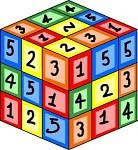## Thursday, November 18, 2010

### MERIDA MIKEY ON GIVING 100%Here is a little something someone sent me that is indisputable mathematical logic. This is a strictly mathematical viewpoint. It goes like this:

What Makes 100%? What does it mean to give MORE than 100%? Ever wonder about those people who say they are giving more than 100%? We have all been to those meetings where someone wants you to give over 100%. How about achieving 103%? What makes up 100% in life?Here's a little mathematical formula that might help you answer these questions.

If:

A B C D E F G H I J K L M N O P Q R S T U V W X Y Z

is represented as:

1 2 3 4 5 6 7 8 9 10 11 12 13 14 15 16 17 18 19 20 21 22 23 24 25 26.

8+1+18+4+23+15+18+11 = 98%

and

11+14+15+23+12+5+4+7+5= 96%

But

1+20+20+9+20+21+4+5 = 100%

And,

2+21+12+12+19+8+9+20 = 103%

AND, look how far ass kissing will take you.

1+19+19+11+9+19+19+9+14+7= 118%

So, one can conclude with mathematical certainty, that While Hard work and Knowledge will get you close, and Attitude will get you there, it’s the Bullshit and Ass kissing that will put you over the top.

P.S. I didn't chek with the internet Gestapo, Snopes, to see if this is true.#### 1 comment:

1.We had an incentive system in the steel mill that was put in place in the 30s, it had straight time pegged at 100% and anything above that was gravy. But the extra time paid was stuck in a time warp from the 60s so our straight time was paid at 15-20 bucks an hour but our incentive was 3 or 4 bucks an hour. I was on a job that in the 60s had about 40 guys doing the work, by the time I fell into that feather bed job it was 6 guys doing the same work as the 40 and those feathers were pretty flat but it paid well. 40 guys' incentive boiled down to 6 guys is a pile of money even at 1966 rates of pay. But the stress almost killed me, a 100% should be good enough for any working man.